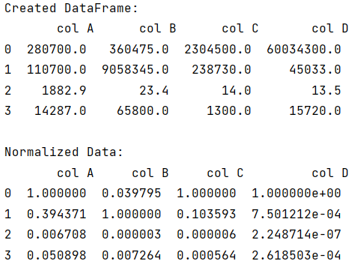# Data Normalization in Pandas

Learn about the Data Normalization in Pandas with example. By Pranit Sharma Last updated : September 23, 2023

Pandas is a special tool that allows us to perform complex manipulations of data effectively and efficiently. Inside pandas, we mainly deal with a dataset in the form of DataFrame. DataFrames are 2-dimensional data structures in pandas. DataFrames consist of rows, columns, and the data.

## Data Normalization in Pandas

Data Normalisation is another important technique in the study of machine learning. It is a process of converting or transforming a numerical column into a standard value by manipulating the data by doing some kind of addition, subtractions, or another operation, also we find absolute values in normalization.

Note

To work with pandas, we need to import pandas package first, below is the syntax:

```import pandas as pd
```

Let us understand with the help of an example,

## Python Program for Data Normalization in Pandas

```# Importing pandas package
import pandas as pd

# Create dictionary
d = {
'col A': [280700, 110700, 1882.9, 14287],
'col B':[360475, 9058345, 23.4, 65800],
'col C':[2304500, 238730, 14.0, 1300],
'col D':[60034300, 45033, 13.5, 15720]
}

# Creating dataframe
df = pd.DataFrame(d)

# Display DataFrame
print("Created DataFrame:\n",df,"\n")

# Normalising data
df_max_scaled = df.copy()

# Applying normalization techniques
for column in df_max_scaled.columns:
df_max_scaled[column] = df_max_scaled[column] / df_max_scaled[column].abs().max()

# Display normalized data
print("Normalized Data:\n",df_max_scaled)
```

### Output

The output of the above program is: